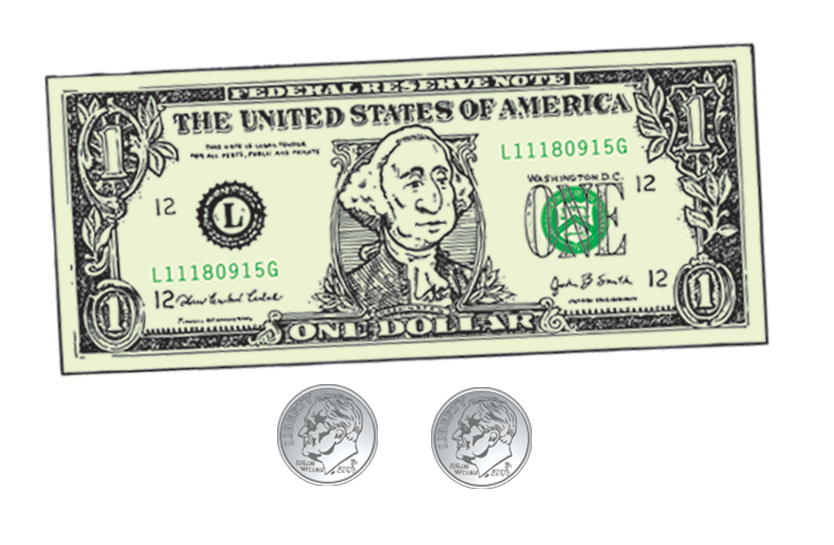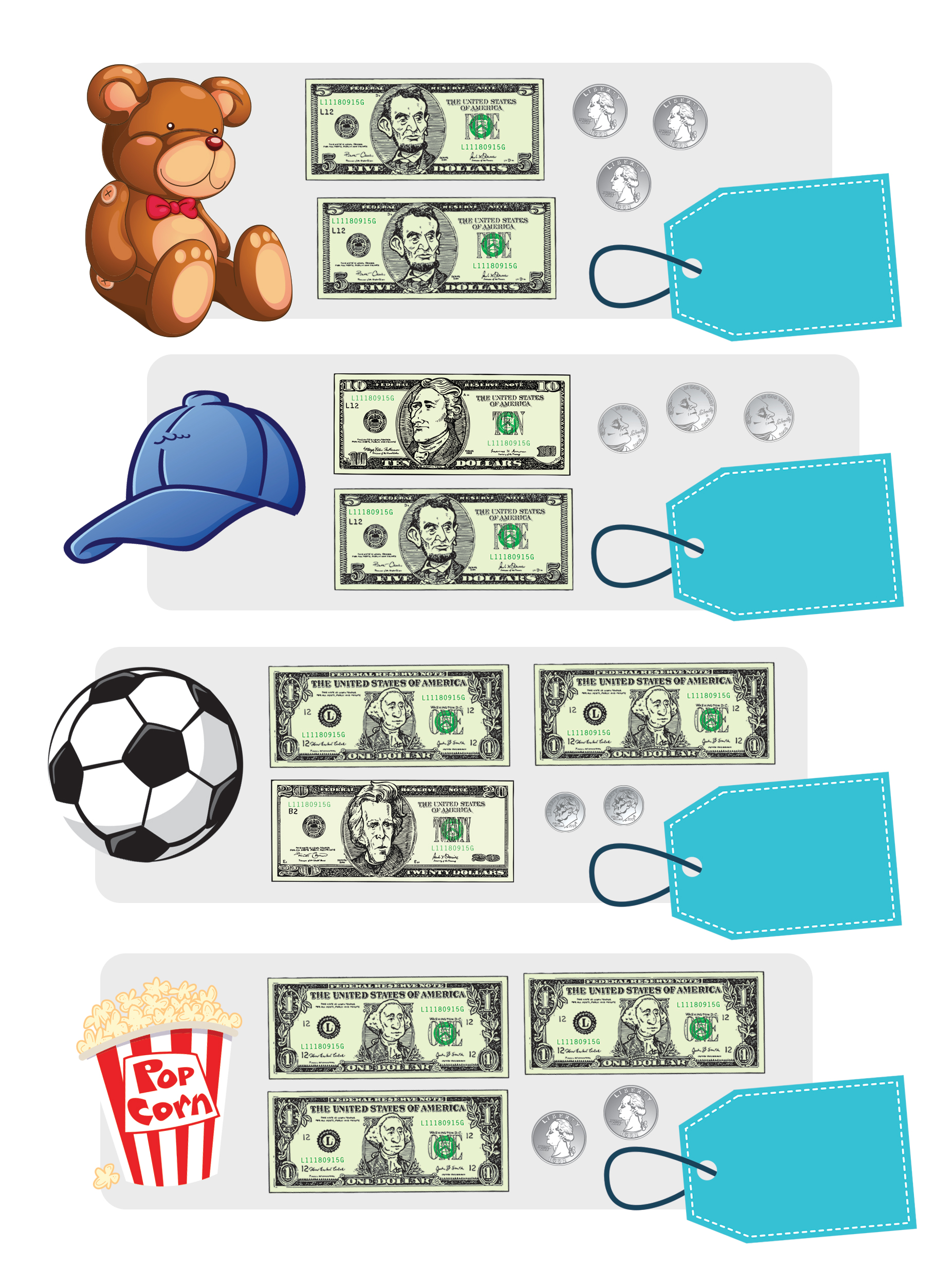Operation: Practice Makes Perfect (AKA: How to write amounts of money)

As a part of your M3 training we’re going to show you how to write amounts of money using a dollar sign (\$) and a decimal point.

Here’s an example:
\$1.20 = 1 dollar and 20 centsNow it’s your turn. Add up the dollars and cents to see if you can figure out the amount that should go on each price tag.M3 Expert tip: If you have coins that add up to more than one dollar, add one dollar to the amount. You can then add the remaining cents.
For example: 5 quarters = \$1.25

Answers: Teddy Bear = \$10.75, Baseball cap = \$15.15, Soccer ball = \$22.20, and popcorn = \$3.50

Back To Money Lab Training Center